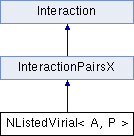ParM  parm A molecular dynamics library
NListedVirial< A, P > Class Template Reference

#include <interaction.hpp>

Inheritance diagram for NListedVirial< A, P >:## Public Member Functions

NListedVirial (sptr< AtomVec > vec, sptr< NeighborList > neighbors)

void set_forces (Box &box)

void set_forces (Box &box, FPairXFunct *)

virtual flt set_forces_get_pressure (Box &box)
Set forces (Atom.f) and return $$P = \sum_{\left<i,j \right>} \vec r_{ij} \cdot \vec F_{ij}$$ at the same time (see pressure()). More...

virtual flt setForcesGetEnergy (Box &box)

virtual flt energy (Box &box)
Potential energy due to this Interaction. More...

virtual flt pressure (Box &box)
Partial pressure due to this Interaction. More...

void add (A atm)

sptr< NeighborListneighbor_list ()Public Member Functions inherited from InteractionPairsX
virtual ~InteractionPairsX ()Public Member Functions inherited from Interaction
virtual Matrix stress (Box &box)
The force-moment tensor for the current simulation: More...

virtual ~Interaction ()

## Constructor & Destructor Documentation

template<class A , class P >
 NListedVirial< A, P >::NListedVirial ( sptr< AtomVec > vec, sptr< NeighborList > neighbors )
inline

## Member Function Documentation

template<class A , class P >
 void NListedVirial< A, P >::add ( A atm )
inline
template<class A , class P >
 virtual flt NListedVirial< A, P >::energy ( Box & box )
inlinevirtual

Potential energy due to this Interaction.

Implements Interaction.

template<class A , class P >
 sptr NListedVirial< A, P >::neighbor_list ( )
inline
template<class A , class P >
 virtual flt NListedVirial< A, P >::pressure ( Box & box )
inlinevirtual

Partial pressure due to this Interaction.

$$P = \sum_{\left<i,j \right>} \vec r_{ij} \cdot \vec F_{ij}$$, or equivalently $$P = \sum_i \vec r_i \cdot \vec F_i$$

Note that the full pressure involves all interactions and temperature, and needs to be normalized by $$\frac{1}{dV}$$ where $$d$$ is the number of dimensions and $$V$$ is the volume.

Implements Interaction.

template<class A , class P >
 void NListedVirial< A, P >::set_forces ( Box & box )
inlinevirtual

Implements Interaction.

template<class A , class P >
 void NListedVirial< A, P >::set_forces ( Box & box, FPairXFunct * funct )
virtual

Implements InteractionPairsX.

template<class A , class P >
 virtual flt NListedVirial< A, P >::set_forces_get_pressure ( Box & box )
inlinevirtual

Set forces (Atom.f) and return $$P = \sum_{\left<i,j \right>} \vec r_{ij} \cdot \vec F_{ij}$$ at the same time (see pressure()).

Reimplemented from Interaction.

template<class A , class P >
 flt NListedVirial< A, P >::setForcesGetEnergy ( Box & box )
virtual

The documentation for this class was generated from the following file: